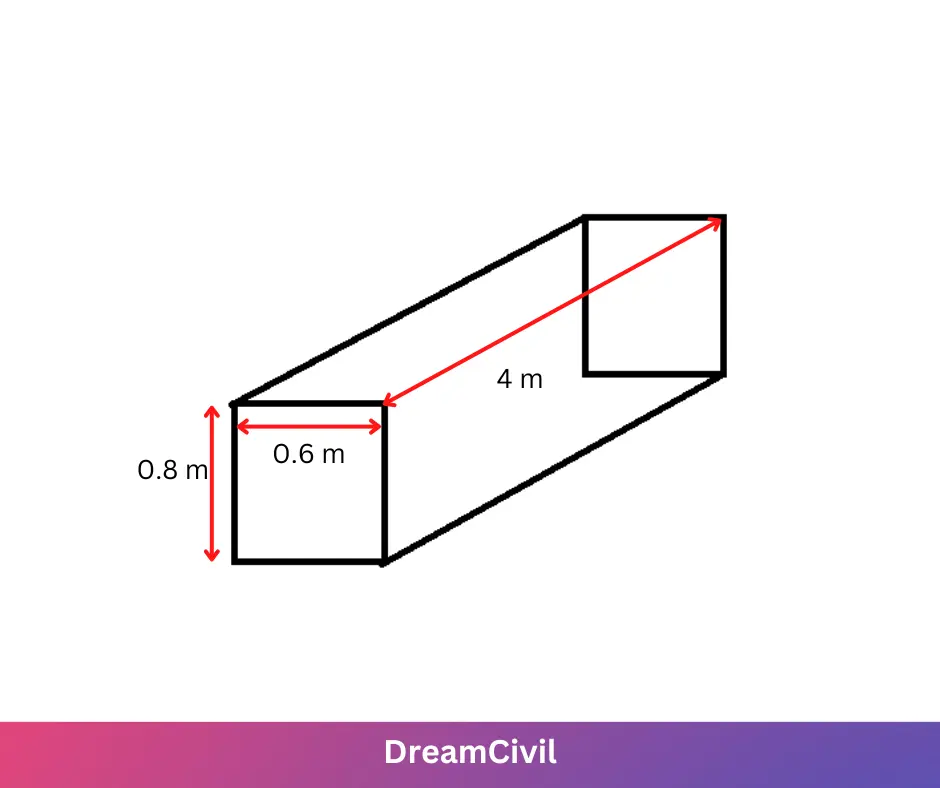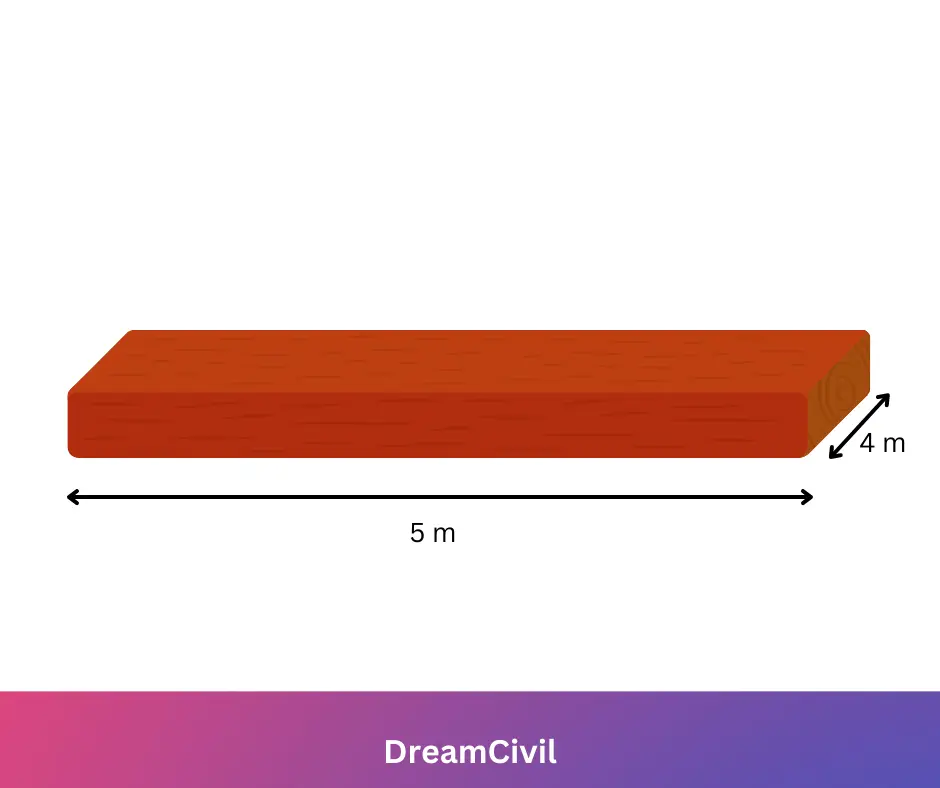Monday, October 2, 2023

# How to Calculate Shuttering Area?

Formwork (also called falsework, centering, and shuttering) may be defined as the timber or other materials which are used to form molds into which concrete is poured at the site.

### 1. Requirements of Good Shuttering

The following are the requirements of good formwork:

a. It should be adequately strong.

b. It should be stiff.

c. It should be smooth.

d. It should provide a good shape at the concrete face.

The following points about formwork are worth noting:

a. The joints made in the formwork should be simple. The joints of the formwork should be preferably tongued and grooved to ensure water-tightness and minimize the possibility of the slurry oozing out.

b. The surface of formwork that is to come in contact with concrete is usually coated with crude oil or soft soap solution, to prevent the possibility of concrete getting stuck to it.

c. All formwork must be so made that it can be easily stripped after concrete sets.

d. The formwork should be so designed and constructed that it can be struck in the desired order.

e. Every effort shall be made to minimize timber wastage during the construction of formwork since the cost of formwork may amount to about 20 % of the cost of some of the jobs.

f. The formwork should be carefully inspected by the Engineer-in-chief and only after making sure that the formwork is properly made, the concreting process must be allowed.

g. The formwork, during concreting, should be continuously in observation for bulges and other signs of failure.

### 2. How to Calculate Shuttering Area?

The shuttering is estimated in terms of Square meters in the rate analysis of shuttering. To estimate the area of shuttering, we must understand how to compute the peripheral length (Perimeter) of any shape.

The perimeter may be defined as the distance around a two-dimensional shape.
For example, the square has four sides each of equal length is “s”
then peripheral length  = s+s+s+s = 4s

#### a. Essential Formula for Computing Shuttering Area

Perimeter of Square : 4S  (S = Length of Side)

Perimeter of Rectangle : 2[L+B]  (L=Length & B = Breadth)

Perimeter of Circle : 2πr  (r = Radius of circle)

Area of Rectangle = Length x Breadth

Area of Square = Side x Side

Each member in a structure either may be Slab or Beam or Column it includes six sides (faces). The shuttering area can be estimated by employing two ways. One is by the formula noted below and another method is by estimating the particular areas of faces.

#### b. Forumla of Shuttering Area

Shuttering Area = Peripheral Length x Depth

### 3. Calculation of Shuttering Area of a Column

Assume a column as shown in the figure. To compute the shuttering area follow these ways:-

For the Column, shuttering is accomplished for four sides and the other two sides (the top of the column is left for supplying concrete, and the base is fixed to ground level). Ignore the top and bottom in the calculation.The side of the column is in a rectangle shape with side length “l” and breadth “b”

Peripheral length of Rectangle = l+b+l+b = 2l+2b

Shuttering area = Peripheral length (Perimeter) x Depth

Peripheral length = 2×0.8+2×0.6 = 1.6+1.2 = 2.8Sq.m

Total Area of Shuttering of a column = 2.8 x 4 = 11.2 Sq.m

The shape of the column is Circular then the formula is employed for estimating the shuttering area.

Shuttering area of Circular Column = 2πr  x Depth

### 4. Calculation of Shuttering Area of a Beam

For Beam, shuttering is done on 5 sides, and the other side (the top side is left to fill concrete)Shuttering area can also be estimated by computing out the particular area of each face as below:

Face 1 : Area of rectangle = L x B = 0.8 x 4 = 3.2
Face 2 : Area of rectangle = L x B = 0.6 x 4 = 2.4
Face 3 : Area of rectangle = L x B = 0.8 x 4 = 3.2
Face 4 : Area of rectangle = L x B = 0.8 x 0.6 = 0.48
Face 5 : Area of rectangle = L x B = 0.8 x 0.6 = 0.48

Total Area of Shuttering = 3.2 + 2.4 + 3.2+ 0.48 +0.48 = 9.76 m²

### 5. Calculation of Shuttering Area of a Slab

The slab lies on a beam, there is no necessity of putting shuttering to the slab on four sides.  Same as the beam & column, the top of the slab is left to supply concrete and for curing. Hence, shuttering is only kept at the base of the slab. The values noted below are used for computing the shuttering of a slab.Shuttering area of Slab =  Bottom area of slab = L x B
Base Area =  5 x 4 = 20 Sq.m

Hope you got an idea of how to calculate shuttering area.He is a founder and lead author of Dream Civil International and his civil engineering research articles has been taken as source by world's top news and educational sites like USA Today, Time, The richest, Wikipedia, etc.
Latest Articles

Related Articles